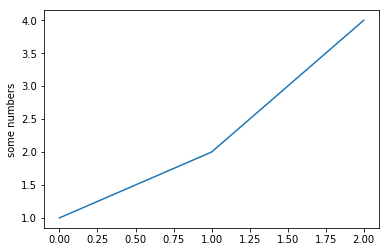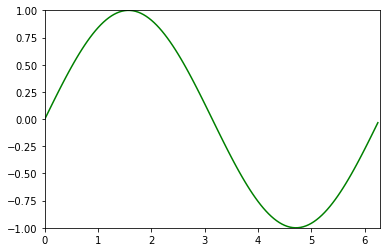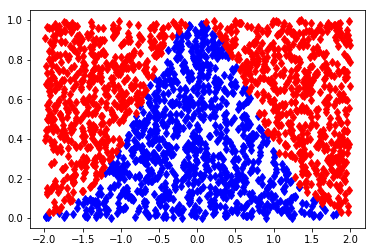# Modules and packaging; numpy¶

## Using modules¶

A python module is non other than a bunch of functions and classes, packed togeather. You can write your own module also, which can be used by others.

The installed modules can be imported easily.

To install a module see this or that.

In :
import sys
print sys.path

['', 'C:\\ProgramData\\Anaconda2\\python27.zip', 'C:\\ProgramData\\Anaconda2\\DLLs', 'C:\\ProgramData\\Anaconda2\\lib', 'C:\\ProgramData\\Anaconda2\\lib\\plat-win', 'C:\\ProgramData\\Anaconda2\\lib\\lib-tk', 'C:\\ProgramData\\Anaconda2', 'C:\\ProgramData\\Anaconda2\\lib\\site-packages', 'C:\\ProgramData\\Anaconda2\\lib\\site-packages\\win32', 'C:\\ProgramData\\Anaconda2\\lib\\site-packages\\win32\\lib', 'C:\\ProgramData\\Anaconda2\\lib\\site-packages\\Pythonwin', 'C:\\ProgramData\\Anaconda2\\lib\\site-packages\\IPython\\extensions', 'C:\\Users\\Gabor\\.ipython']


sys is a built-in module, it is installed by default. This module can access system files and variables (for example command line arguments).

The sys.path is a variable containing various directory paths.

You can import only one function from a module of the whole module.

In :
import math

print math.pi

from itertools import permutations
for p in permutations(['A','B','C']):
print p

3.14159265359
('A', 'B', 'C')
('A', 'C', 'B')
('B', 'A', 'C')
('B', 'C', 'A')
('C', 'A', 'B')
('C', 'B', 'A')


### Relative import¶

You can import files in the current directory.

In :
from lab11 import Node
print Node("3+4*5")

<lab11.Node object at 0x0000000004E2BE10>


## numpy basics¶

Numpy is a module for numerical calculations. It can handle vectors, matrices, arrays and perform linear algebraic calculations, random number generation.

If you have Anaconda then it is installed by default.

You can import a module with an alternative name, just to make it shorter.

 import numpy as np

The main object of numpy is ndarray, short for n-dimensional array, you can create arrays with the numpy.array function.

In :
import numpy as np
x = np.arange(1,-1,-0.1)
y = np.array([[1,2,3],[1,2,4]])

In :
print x.dtype
print y.dtype
print x.shape
print y.shape
print x.ndim
print y.ndim
print x
print y

float64
int32
(20L,)
(2L, 3L)
1
2
[ 1.00000000e+00  9.00000000e-01  8.00000000e-01  7.00000000e-01
6.00000000e-01  5.00000000e-01  4.00000000e-01  3.00000000e-01
2.00000000e-01  1.00000000e-01  2.22044605e-16 -1.00000000e-01
-2.00000000e-01 -3.00000000e-01 -4.00000000e-01 -5.00000000e-01
-6.00000000e-01 -7.00000000e-01 -8.00000000e-01 -9.00000000e-01]
[[1 2 3]
[1 2 4]]


You can perform elementwise operations (+ - * /) if the arrays are compatible.

In :
a = np.array( [20,30,40,50] )
b = np.arange( 4 )
print a+b
print a-b
print b / (b+1.0)
print np.sin(b)

[20 31 42 53]
[20 29 38 47]
[0.         0.5        0.66666667 0.75      ]
[0.         0.84147098 0.90929743 0.14112001]


You can add a number to an array which means adding the same number to all of the elements. Same for multiplication and other operations.

In :
b = np.arange(10)
print b
print b ** 2
print b + 10
print b % 3 == 1

[0 1 2 3 4 5 6 7 8 9]
[ 0  1  4  9 16 25 36 49 64 81]
[10 11 12 13 14 15 16 17 18 19]
[False  True False False  True False False  True False False]


The matrix dot product is not the * operator!

In :
A=np.arange(2,6).reshape(2,2)
B=np.arange(3,-1,-1).reshape(2,2)
print A
print B
print A*B
print A.dot(B)

[[2 3]
[4 5]]
[[3 2]
[1 0]]
[[6 6]
[4 0]]
[[ 9  4]
[17  8]]


Note that the reshape can restructure the elements into a different array. The number of elements should not change.

The dot raises an error if the operands are not compatible.

You can use the normal indexing.

In :
x = np.arange(15).reshape(3,5)
print x
print x[0:2]

[[ 0  1  2  3  4]
[ 5  6  7  8  9]
[10 11 12 13 14]]
[[0 1 2 3 4]
[5 6 7 8 9]]


Or you can take certain columns:

In :
print x[:,3]
print x[2,:3]

[ 3  8 13]
[10 11 12]


You can use a list of indices which slices the corresponding rows (or columns).

In :
a = np.arange(12)**2
i = np.array( [ 1,1,3,8,5 ] )
print a
print a[i]
A = np.arange(15).reshape(3,5)
print
print A
print A[:, [0,2]]

[  0   1   4   9  16  25  36  49  64  81 100 121]
[ 1  1  9 64 25]

[[ 0  1  2  3  4]
[ 5  6  7  8  9]
[10 11 12 13 14]]
[[ 0  2]
[ 5  7]
[10 12]]


You can call a numpy function with an array parameter which performs (mostly) elementwise operation.

Numpy can calculate mean and standard deviation.

In :
x = np.log(np.arange(2,10,0.5))
print x
print x.sum()
print x.mean()
print x.std()

[0.69314718 0.91629073 1.09861229 1.25276297 1.38629436 1.5040774
1.60943791 1.70474809 1.79175947 1.87180218 1.94591015 2.01490302
2.07944154 2.14006616 2.19722458 2.2512918 ]
26.457769829012314
1.6536106143132696
0.4600673068044455


To make an array filled with zeros or ones you can call zeros or ones, similar to MatLab. The identity matrix is eye.

In :
print np.zeros([4,3])
print np.ones([4,1])
print np.eye(4)

[[0. 0. 0.]
[0. 0. 0.]
[0. 0. 0.]
[0. 0. 0.]]
[[1.]
[1.]
[1.]
[1.]]
[[1. 0. 0. 0.]
[0. 1. 0. 0.]
[0. 0. 1. 0.]
[0. 0. 0. 1.]]


You can generate random numbers or array of numbers.

In :
np.random.rand(3,4)

Out:
array([[0.45424234, 0.49949172, 0.48945174, 0.89843896],
[0.05093053, 0.61863866, 0.87536574, 0.47432188],
[0.31387043, 0.42734447, 0.3104697 , 0.37358285]])

You can generate uniform numbers drawn from $[-2, 2]$ in two ways:

In :
print np.random.rand(10)*4-2
print np.random.uniform(size=10, high=2, low=-2)

[-0.89928203 -1.22141331 -1.07179354 -0.05271209  0.17675864 -1.75561769
-0.17835643 -1.2692697   0.60508815  1.96248121]
[-0.45413086 -1.81227906 -1.76056501  1.03348617  0.8038665   0.02720191
1.2014611   1.31753676  0.52944959 -0.80288664]


## Plot¶

The matplotlib module can plot functions.

In :
%matplotlib inline
import matplotlib
import matplotlib.pyplot as plt


A simple plot first. If you don't specify the $x$ values, the range $[0, 1, 2 \ldots]$ is used instead.

In :
plt.plot([1,2,4])
plt.ylabel('some numbers')
plt.show()These libraries cannot calculate symbolically, like Sage, just makes a series of lines between the plotted points.

Let's plot a sine curve.

In :
plt.plot(np.arange(0,2*np.pi,0.05), np.sin(np.arange(0,2*np.pi,0.05)), 'g')
plt.axis([0,2*np.pi,-1,1])
plt.show()### Monte-Carlo¶

Monte-Carlo simulation is an easy but not too fast way to estimate an integral.

To calculate $\int_{-2}^2e^{-x^2}\,\mathrm{d}x$ you draw random points in the rectangle $[-2,2]\times[0,1]$ and count how many points fell under the graph: $e^{-x^2} > y$.

The ratio of the points under the curve times the size of the rectangle is an approximation of the area.

In :
X = np.random.rand(500000,2)
X[:,0] = X[:,0]*4-2
J = np.where(X[:,1] < np.exp(-X[:,0]**2))
print len(J) / 500000.0 * 4

1.758016


In picture:

In :
Xp = X[:2000]
Ip = [i for i in range(len(Xp)) if i in J]
Inp = [i for i in range(len(Xp)) if i not in J]
plt.plot(Xp[Ip,0],Xp[Ip,1], 'bd', Xp[Inp,0],Xp[Inp,1], 'rd')
plt.show()In [ ]: